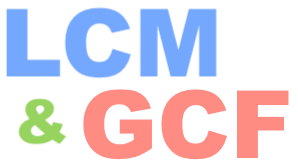What is the LCM and GCF of 13 and 42?The question "What is the LCM and GCF of 13 and 42?" can be split into two questions: "What is the LCM of 13 and 42?" and "What is the GCF of 13 and 42?"

In the question "What is the LCM and GCF of 13 and 42?", LCM is the abbreviation of Least Common Multiple and GCF is the abbreviation of Greatest Common Factor.

To find the LCM, we first list the multiples of 13 and 42 and then we find the smallest multiple they have in common. To find the multiples of any number, you simply multiply the number by 1, then by 2, then by 3 and so on. Here is the beginning list of multiples of 13 and 42:

Multiples of 13: 13, 26, 39, 52, 65, 78, etc.

Multiples of 42: 42, 84, 126, 168, 210, 252, etc.

The least multiple on the two lists that they have in common is the LCM of 13 and 42. Therefore, the LCM of 13 and 42 is 546.

To find the GCF, we first list the factors of 13 and 42 and then we find the largest factor they have in common. The factors of any number, are all the numbers that you can evenly divide into that number.

In other words, the factors of 13 are all the numbers that can evenly divide into 13, and the factors of 42 are all the numbers that can evenly divide into 42. Here are the factors for 13 and 42:

Factors of 13: 1 and 13.

Factors of 42: 1, 2, 3, 6, 7, 14, 21, and 42.

The greatest factor on the two lists that they have in common is the GCF of 13 and 42. Therefore, the GCF of 13 and 42 is 1.

In summary, the answer to the question "What is the LCM and GCF of 13 and 42?" is 546 and 1.

LCM and GCF Calculator
Do you need the LCM and GCF for another set of numbers? No problem, please enter them below:

&

What is the LCM and GCF of 13 and 43?
Here is the next LCM and GCF question on our list that we have tackled!